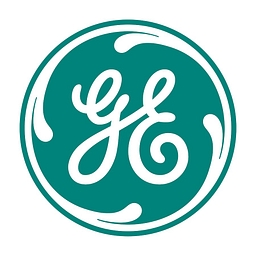# The Best Dogs of the Dow Jones As Of February 2012

+56.29%
Upside
75.25
Market
118
TrefisGE
General Electric

This article was submitted by Dividend Yield using our Trefis Contributors tool

Here is an updated sheet of the 10 best Dogs of the Dow. Such stocks have the lowest expected price to earnings ratio and highest dividend yield within the Dow Jones Index. The ten cheapest stocks of the Dow Jones have an average dividend yield of 3.72 percent as well as a forward P/E ratio of 11.69. The average P/B ratio amounts to 2.74 and P/S ratio is 1.89. The two best results are still AT&T (T) and Verizon (VZ). Both companies come from the domestic telecom services industry and have a yield over 5 percent. The second strongly represented industry is the major drug manufacturing industry, covering the following positions. But the best rated stocks are Kraft Foods (KFT) and Pfizer (PFE).

These are the best dogs by current P/E ratio:

Relevant Articles

1. General Electric Company (GE) has a market capitalization of \$203.12 billion. The company employs 300,000 people, generates revenues of \$147,300.00 million and has a net income of \$14,366.00 million. The firm’s earnings before interest, taxes, depreciation and amortization (EBITDA) amounts to \$20,098.00 million. Because of these figures, the EBITDA margin is 13.64 percent (operating margin 13.64 percent and the net profit margin finally 9.75 percent).

The total debt representing 63.21 percent of the company’s assets and the total debt in relation to the equity amounts to 389.52 percent. Due to the financial situation, a return on equity of 11.09 percent was realized. Twelve trailing months earnings per share reached a value of \$1.23. Last fiscal year, the company paid \$0.61 in form of dividends to shareholders.

Here are the price ratios of the company: The P/E ratio is 15.68, P/S ratio 1.38 and P/B ratio 1.75. Dividend Yield: 3.53 percent. The beta ratio is 1.60.

2. E I Du Pont De Nemours (DD) has a market capitalization of \$47.54 billion. The company employs 70,000 people, generates revenues of \$38,719.00 million and has a net income of \$3,510.00 million. The firm’s earnings before interest, taxes, depreciation and amortization (EBITDA) amounts to \$6,289.00 million. Because of these figures, the EBITDA margin is 16.24 percent (operating margin 11.06 percent and the net profit margin finally 9.07 percent).

The total debt representing 25.89 percent of the company’s assets and the total debt in relation to the equity amounts to 146.08 percent. Due to the financial situation, a return on equity of 39.82 percent was realized. Twelve trailing months earnings per share reached a value of \$3.68. Last fiscal year, the company paid \$1.64 in form of dividends to shareholders.

Here are the price ratios of the company: The P/E ratio is 13.86, P/S ratio 1.23 and P/B ratio 5.65. Dividend Yield: 3.22 percent. The beta ratio is 1.46.

3. Intel Corporation (INTC) has a market capitalization of \$133.39 billion. The company employs 100,100 people, generates revenues of \$53,999.00 million and has a net income of \$12,942.00 million. The firm’s earnings before interest, taxes, depreciation and amortization (EBITDA) amounts to \$23,409.00 million. Because of these figures, the EBITDA margin is 43.35 percent (operating margin 32.12 percent and the net profit margin finally 23.97 percent).

The total debt representing 10.31 percent of the company’s assets and the total debt in relation to the equity amounts to 15.97 percent. Due to the financial situation, a return on equity of 27.15 percent was realized. Twelve trailing months earnings per share reached a value of \$2.40. Last fiscal year, the company paid \$0.78 in form of dividends to shareholders.

Here are the price ratios of the company: The P/E ratio is 11.14, P/S ratio 2.47 and P/B ratio 2.91. Dividend Yield: 3.15 percent. The beta ratio is 1.09.

Take a closer look at the full table of the best dogs of the dow. The average P/E ratio amounts to 20.10 and the forward P/E ratio is 11.69. The dividend yield has a value of 3.72 percent. P/B ratio is 2.4 and P/S ratio 1.89. The operating margin amounts to 16.72 percent.